# Math Gr4 Ch12

13. Sep 2009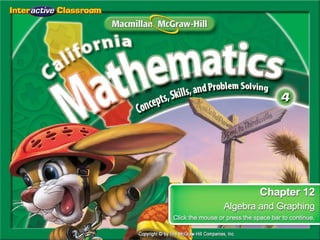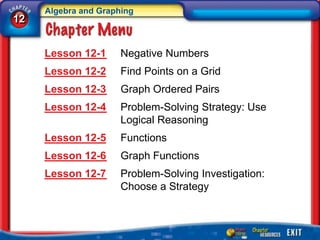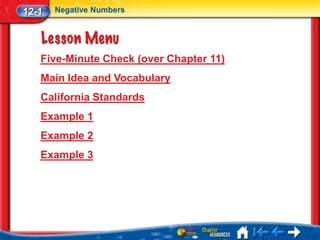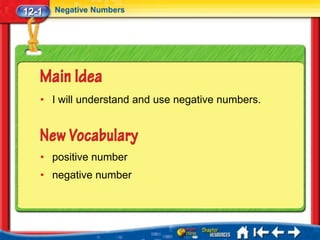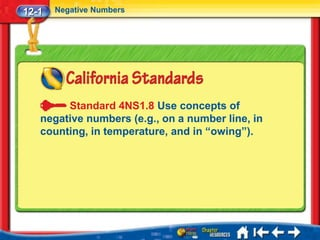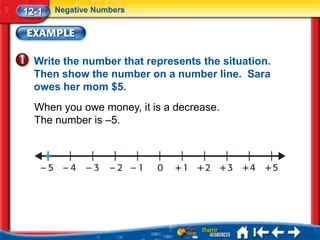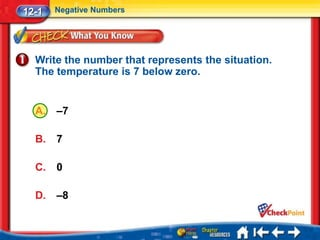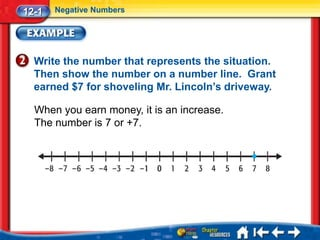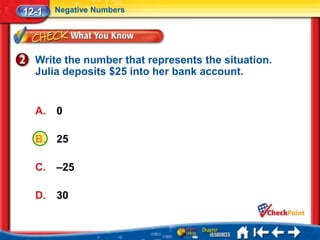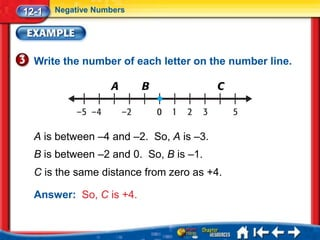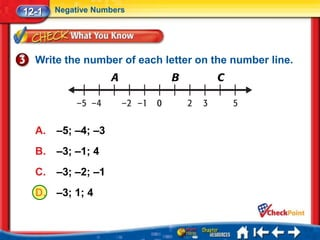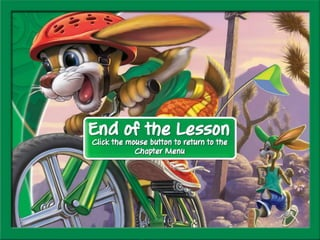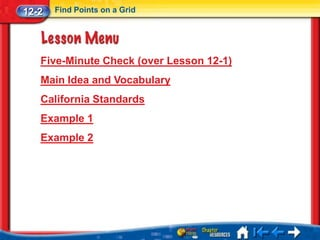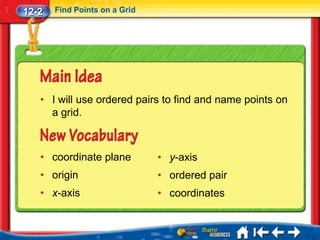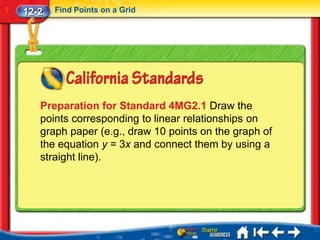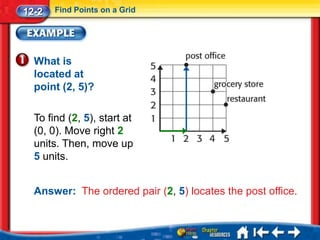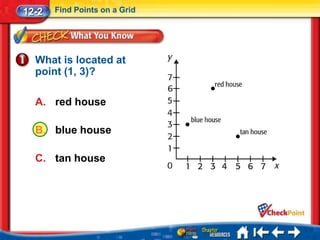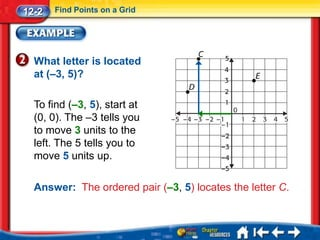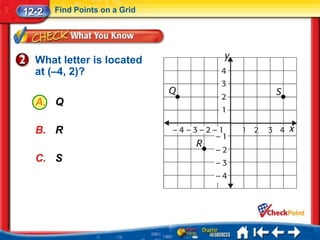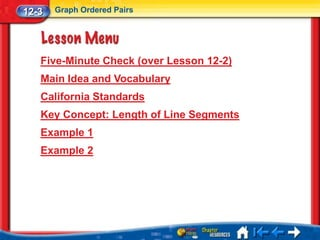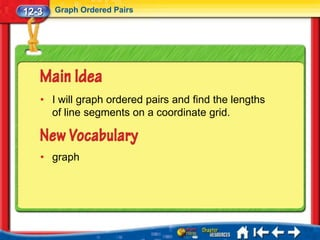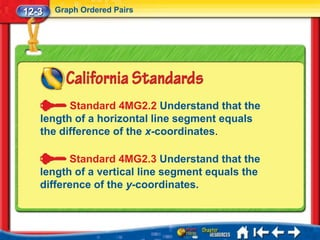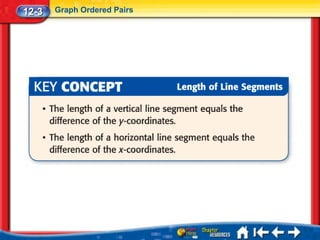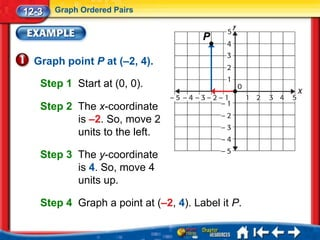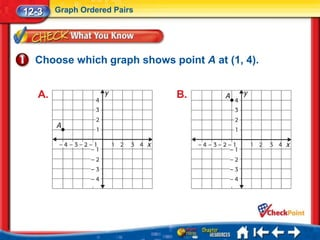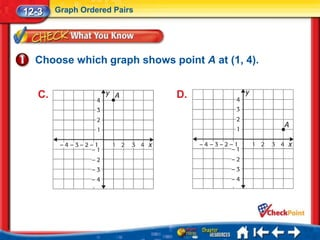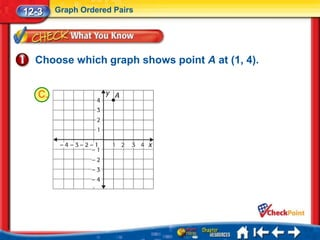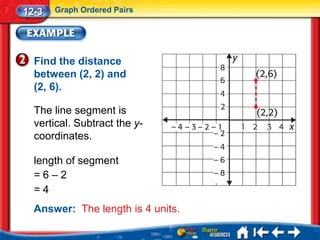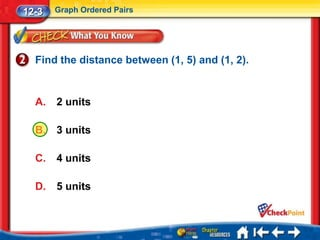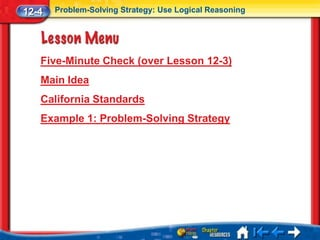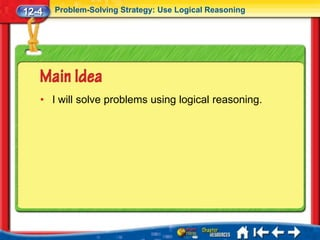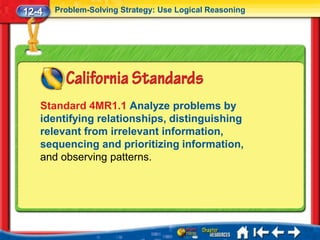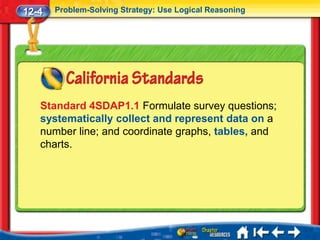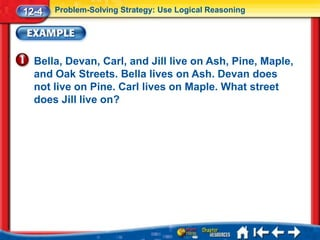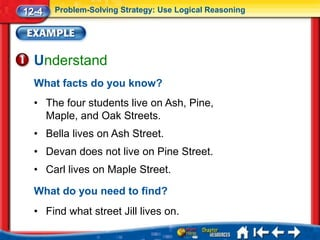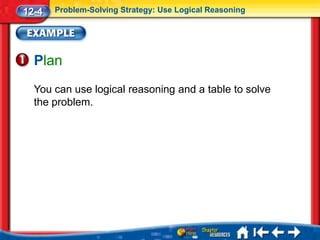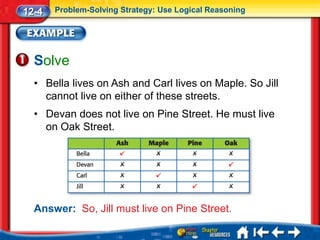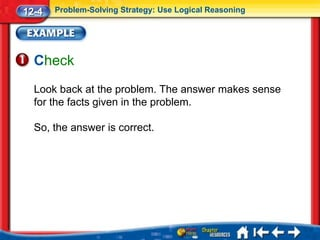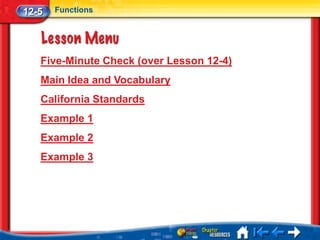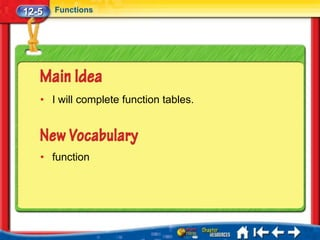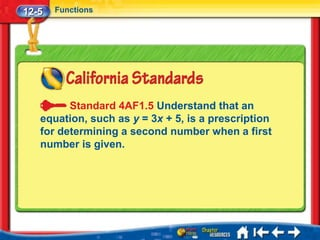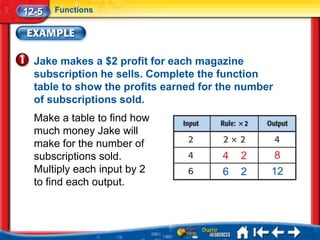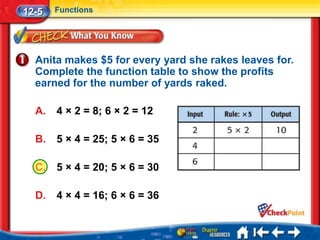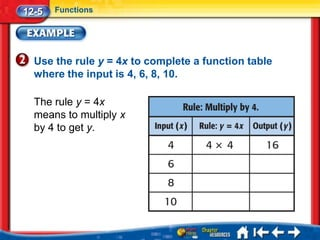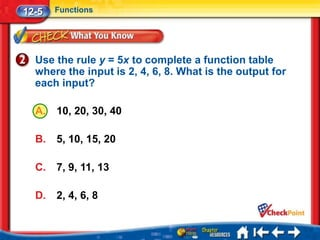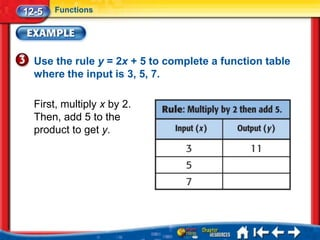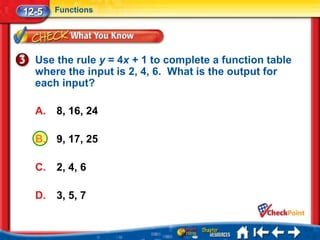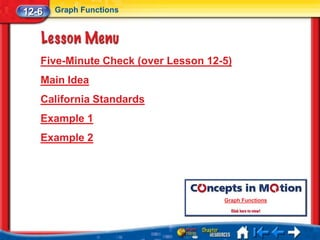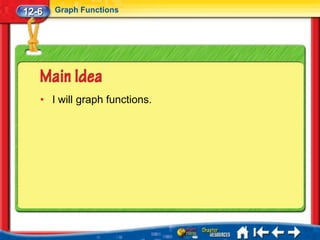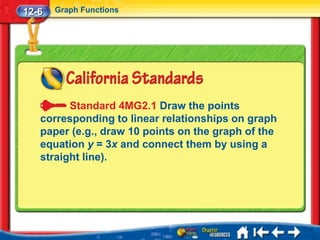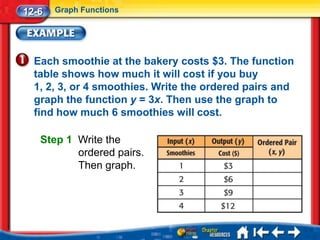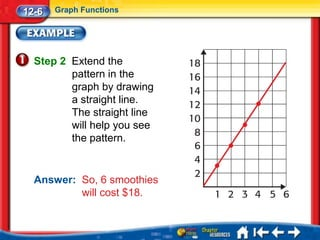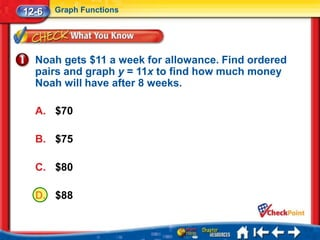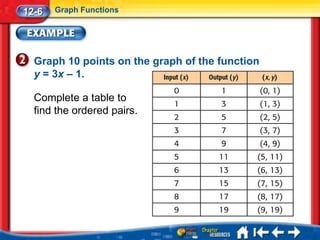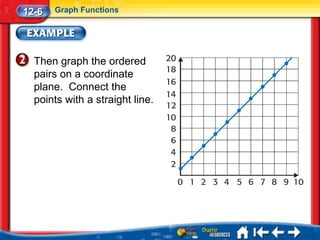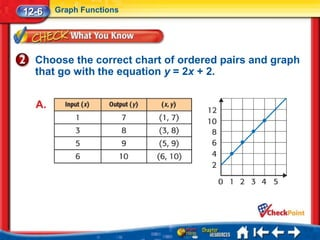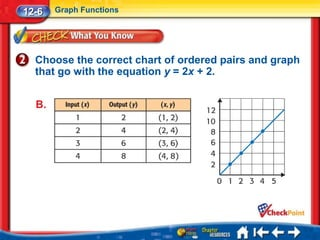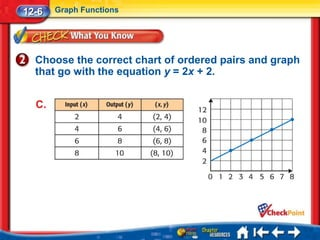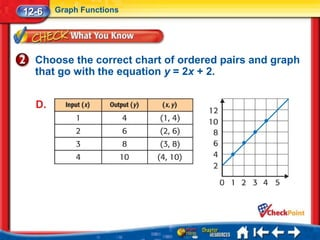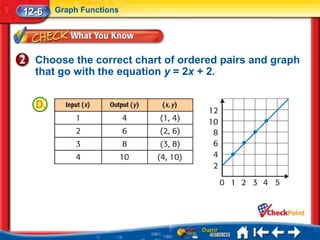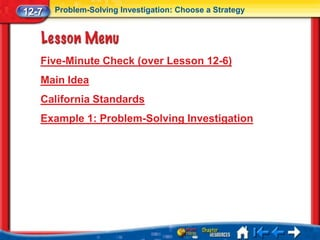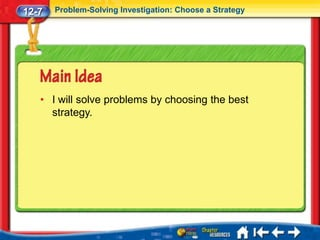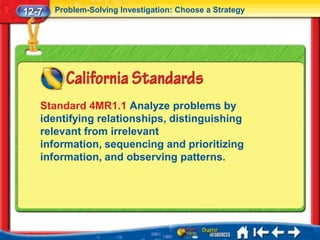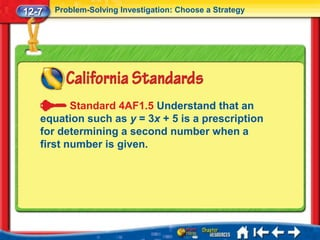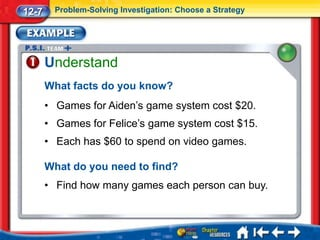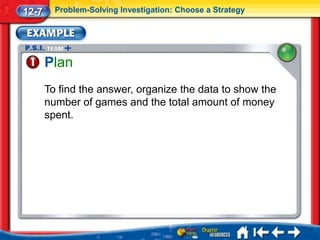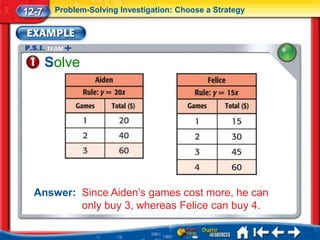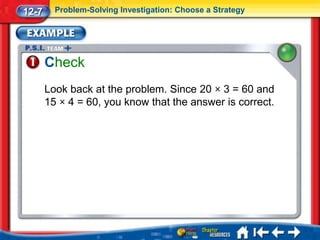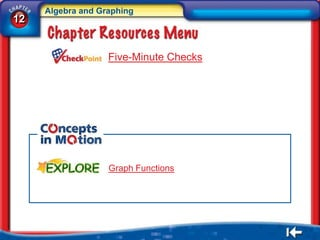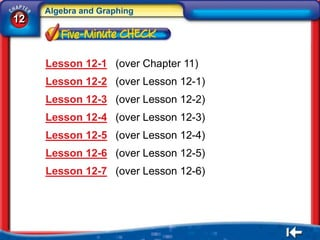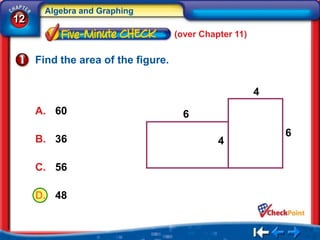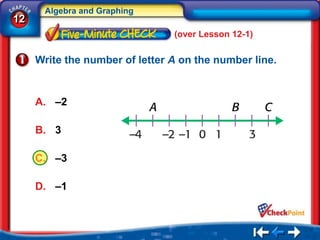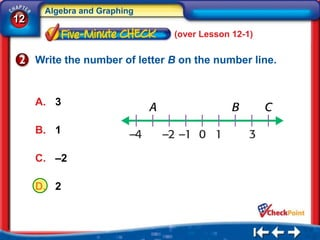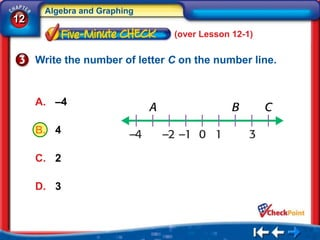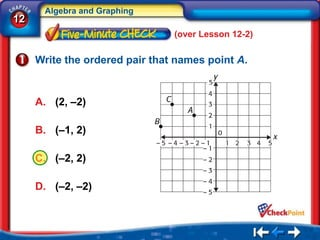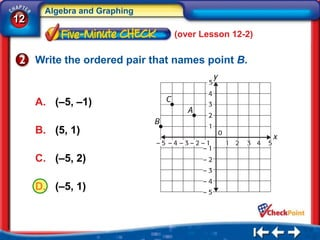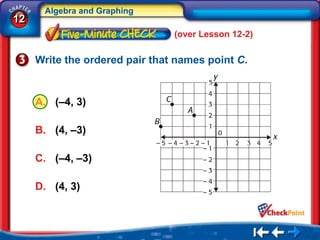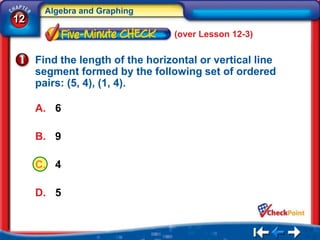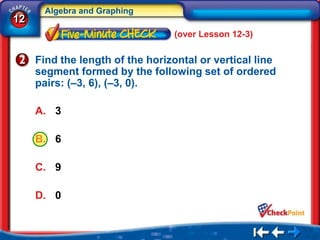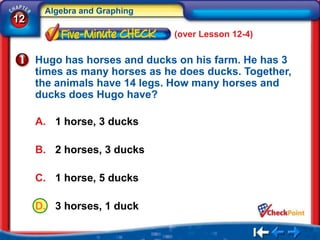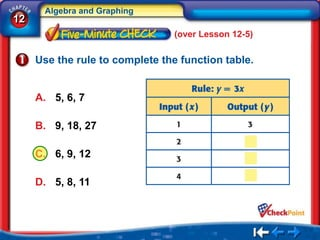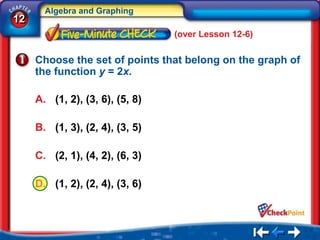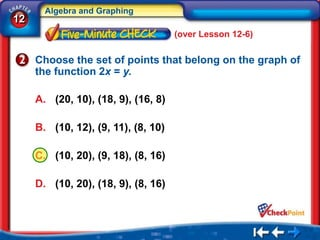1 von 91

### Math Gr4 Ch12

• 1. Chapter 12 Algebra and Graphing Click the mouse or press the space bar to continue.
• 2. Algebra and Graphing 12 Lesson 12-1 Negative Numbers Lesson 12-2 Find Points on a Grid Lesson 12-3 Graph Ordered Pairs Lesson 12-4 Problem-Solving Strategy: Use Logical Reasoning Lesson 12-5 Functions Lesson 12-6 Graph Functions Lesson 12-7 Problem-Solving Investigation: Choose a Strategy
• 3. 12-1 Negative Numbers Five-Minute Check (over Chapter 11) Main Idea and Vocabulary California Standards Example 1 Example 2 Example 3
• 4. 12-1 Negative Numbers • I will understand and use negative numbers. • positive number • negative number
• 5. 12-1 Negative Numbers Standard 4NS1.8 Use concepts of negative numbers (e.g., on a number line, in counting, in temperature, and in “owing”).
• 6. 12-1 Negative Numbers Write the number that represents the situation. Then show the number on a number line. Sara owes her mom \$5. When you owe money, it is a decrease. The number is –5.
• 7. 12-1 Negative Numbers Write the number that represents the situation. The temperature is 7 below zero. A. –7 B. 7 C. 0 D. –8
• 8. 12-1 Negative Numbers Write the number that represents the situation. Then show the number on a number line. Grant earned \$7 for shoveling Mr. Lincoln’s driveway. When you earn money, it is an increase. The number is 7 or +7.
• 9. 12-1 Negative Numbers Write the number that represents the situation. Julia deposits \$25 into her bank account. A. 0 B. 25 C. –25 D. 30
• 10. 12-1 Negative Numbers Write the number of each letter on the number line. A is between –4 and –2. So, A is –3. B is between –2 and 0. So, B is –1. C is the same distance from zero as +4. Answer: So, C is +4.
• 11. 12-1 Negative Numbers Write the number of each letter on the number line. A. –5; –4; –3 B. –3; –1; 4 C. –3; –2; –1 D. –3; 1; 4
• 13. 12-2 Find Points on a Grid Five-Minute Check (over Lesson 12-1) Main Idea and Vocabulary California Standards Example 1 Example 2
• 14. 12-2 Find Points on a Grid • I will use ordered pairs to find and name points on a grid. • coordinate plane • y-axis • origin • ordered pair • x-axis • coordinates
• 15. 12-2 Find Points on a Grid Preparation for Standard 4MG2.1 Draw the points corresponding to linear relationships on graph paper (e.g., draw 10 points on the graph of the equation y = 3x and connect them by using a straight line).
• 16. 12-2 Find Points on a Grid What is located at point (2, 5)? To find (2, 5), start at (0, 0). Move right 2 units. Then, move up 5 units. Answer: The ordered pair (2, 5) locates the post office.
• 17. 12-2 Find Points on a Grid What is located at point (1, 3)? A. red house B. blue house C. tan house
• 18. 12-2 Find Points on a Grid What letter is located at (–3, 5)? To find (–3, 5), start at (0, 0). The –3 tells you to move 3 units to the left. The 5 tells you to move 5 units up. Answer: The ordered pair (–3, 5) locates the letter C.
• 19. 12-2 Find Points on a Grid What letter is located at (–4, 2)? A. Q B. R C. S
• 21. 12-3 Graph Ordered Pairs Five-Minute Check (over Lesson 12-2) Main Idea and Vocabulary California Standards Key Concept: Length of Line Segments Example 1 Example 2
• 22. 12-3 Graph Ordered Pairs • I will graph ordered pairs and find the lengths of line segments on a coordinate grid. • graph
• 23. 12-3 Graph Ordered Pairs Standard 4MG2.2 Understand that the length of a horizontal line segment equals the difference of the x-coordinates. Standard 4MG2.3 Understand that the length of a vertical line segment equals the difference of the y-coordinates.
• 24. 12-3 Graph Ordered Pairs
• 25. 12-3 Graph Ordered Pairs P Graph point P at (–2, 4). Step 1 Start at (0, 0). Step 2 The x-coordinate is –2. So, move 2 units to the left. Step 3 The y-coordinate is 4. So, move 4 units up. Step 4 Graph a point at (–2, 4). Label it P.
• 26. 12-3 Graph Ordered Pairs Choose which graph shows point A at (1, 4). A. B.
• 27. 12-3 Graph Ordered Pairs Choose which graph shows point A at (1, 4). C. D.
• 28. 12-3 Graph Ordered Pairs Choose which graph shows point A at (1, 4). C.
• 29. 12-3 Graph Ordered Pairs Find the distance between (2, 2) and (2, 6). The line segment is vertical. Subtract the y- coordinates. length of segment =6–2 =4 Answer: The length is 4 units.
• 30. 12-3 Graph Ordered Pairs Find the distance between (1, 5) and (1, 2). A. 2 units B. 3 units C. 4 units D. 5 units
• 32. 12-4 Problem-Solving Strategy: Use Logical Reasoning Five-Minute Check (over Lesson 12-3) Main Idea California Standards Example 1: Problem-Solving Strategy
• 33. 12-4 Problem-Solving Strategy: Use Logical Reasoning • I will solve problems using logical reasoning.
• 34. 12-4 Problem-Solving Strategy: Use Logical Reasoning Standard 4MR1.1 Analyze problems by identifying relationships, distinguishing relevant from irrelevant information, sequencing and prioritizing information, and observing patterns.
• 35. 12-4 Problem-Solving Strategy: Use Logical Reasoning Standard 4SDAP1.1 Formulate survey questions; systematically collect and represent data on a number line; and coordinate graphs, tables, and charts.
• 36. 12-4 Problem-Solving Strategy: Use Logical Reasoning Bella, Devan, Carl, and Jill live on Ash, Pine, Maple, and Oak Streets. Bella lives on Ash. Devan does not live on Pine. Carl lives on Maple. What street does Jill live on?
• 37. 12-4 Problem-Solving Strategy: Use Logical Reasoning Understand What facts do you know? • The four students live on Ash, Pine, Maple, and Oak Streets. • Bella lives on Ash Street. • Devan does not live on Pine Street. • Carl lives on Maple Street. What do you need to find? • Find what street Jill lives on.
• 38. 12-4 Problem-Solving Strategy: Use Logical Reasoning Plan You can use logical reasoning and a table to solve the problem.
• 39. 12-4 Problem-Solving Strategy: Use Logical Reasoning Solve • Bella lives on Ash and Carl lives on Maple. So Jill cannot live on either of these streets. • Devan does not live on Pine Street. He must live on Oak Street. Answer: So, Jill must live on Pine Street.
• 40. 12-4 Problem-Solving Strategy: Use Logical Reasoning Check Look back at the problem. The answer makes sense for the facts given in the problem. So, the answer is correct.
• 42. 12-5 Functions Five-Minute Check (over Lesson 12-4) Main Idea and Vocabulary California Standards Example 1 Example 2 Example 3
• 43. 12-5 Functions • I will complete function tables. • function
• 44. 12-5 Functions Standard 4AF1.5 Understand that an equation, such as y = 3x + 5, is a prescription for determining a second number when a first number is given.
• 45. 12-5 Functions Jake makes a \$2 profit for each magazine subscription he sells. Complete the function table to show the profits earned for the number of subscriptions sold. Make a table to find how much money Jake will make for the number of subscriptions sold. 4 2 8 Multiply each input by 2 6 2 12 to find each output.
• 46. 12-5 Functions Anita makes \$5 for every yard she rakes leaves for. Complete the function table to show the profits earned for the number of yards raked. A. 4 × 2 = 8; 6 × 2 = 12 B. 5 × 4 = 25; 5 × 6 = 35 C. 5 × 4 = 20; 5 × 6 = 30 D. 4 × 4 = 16; 6 × 6 = 36
• 47. 12-5 Functions Use the rule y = 4x to complete a function table where the input is 4, 6, 8, 10. The rule y = 4x means to multiply x by 4 to get y.
• 48. 12-5 Functions Use the rule y = 5x to complete a function table where the input is 2, 4, 6, 8. What is the output for each input? A. 10, 20, 30, 40 B. 5, 10, 15, 20 C. 7, 9, 11, 13 D. 2, 4, 6, 8
• 49. 12-5 Functions Use the rule y = 2x + 5 to complete a function table where the input is 3, 5, 7. First, multiply x by 2. Then, add 5 to the product to get y.
• 50. 12-5 Functions Use the rule y = 4x + 1 to complete a function table where the input is 2, 4, 6. What is the output for each input? A. 8, 16, 24 B. 9, 17, 25 C. 2, 4, 6 D. 3, 5, 7
• 52. 12-6 Graph Functions Five-Minute Check (over Lesson 12-5) Main Idea California Standards Example 1 Example 2 Graph Functions
• 53. 12-6 Graph Functions • I will graph functions.
• 54. 12-6 Graph Functions Standard 4MG2.1 Draw the points corresponding to linear relationships on graph paper (e.g., draw 10 points on the graph of the equation y = 3x and connect them by using a straight line).
• 55. 12-6 Graph Functions Each smoothie at the bakery costs \$3. The function table shows how much it will cost if you buy 1, 2, 3, or 4 smoothies. Write the ordered pairs and graph the function y = 3x. Then use the graph to find how much 6 smoothies will cost. Step 1 Write the ordered pairs. Then graph.
• 56. 12-6 Graph Functions Step 2 Extend the pattern in the graph by drawing a straight line. The straight line will help you see the pattern. Answer: So, 6 smoothies will cost \$18.
• 57. 12-6 Graph Functions Noah gets \$11 a week for allowance. Find ordered pairs and graph y = 11x to find how much money Noah will have after 8 weeks. A. \$70 B. \$75 C. \$80 D. \$88
• 58. 12-6 Graph Functions Graph 10 points on the graph of the function y = 3x – 1. Complete a table to find the ordered pairs.
• 59. 12-6 Graph Functions Then graph the ordered pairs on a coordinate plane. Connect the points with a straight line.
• 60. 12-6 Graph Functions Choose the correct chart of ordered pairs and graph that go with the equation y = 2x + 2. A.
• 61. 12-6 Graph Functions Choose the correct chart of ordered pairs and graph that go with the equation y = 2x + 2. B.
• 62. 12-6 Graph Functions Choose the correct chart of ordered pairs and graph that go with the equation y = 2x + 2. C.
• 63. 12-6 Graph Functions Choose the correct chart of ordered pairs and graph that go with the equation y = 2x + 2. D.
• 64. 12-6 Graph Functions Choose the correct chart of ordered pairs and graph that go with the equation y = 2x + 2. D.
• 66. 12-7 Problem-Solving Investigation: Choose a Strategy Five-Minute Check (over Lesson 12-6) Main Idea California Standards Example 1: Problem-Solving Investigation
• 67. 12-7 Problem-Solving Investigation: Choose a Strategy • I will solve problems by choosing the best strategy.
• 68. 12-7 Problem-Solving Investigation: Choose a Strategy Standard 4MR1.1 Analyze problems by identifying relationships, distinguishing relevant from irrelevant information, sequencing and prioritizing information, and observing patterns.
• 69. 12-7 Problem-Solving Investigation: Choose a Strategy Standard 4AF1.5 Understand that an equation such as y = 3x + 5 is a prescription for determining a second number when a first number is given.
• 70. 12-7 Problem-Solving Investigation: Choose a Strategy AIDEN: I just got a new video game system. Games cost \$20. Felice has the older version of the video game system. Her games cost \$15. How many video games can we each buy if we each have \$60? YOUR MISSION: Find out how many games each person can buy.
• 71. 12-7 Problem-Solving Investigation: Choose a Strategy Understand What facts do you know? • Games for Aiden’s game system cost \$20. • Games for Felice’s game system cost \$15. • Each has \$60 to spend on video games. What do you need to find? • Find how many games each person can buy.
• 72. 12-7 Problem-Solving Investigation: Choose a Strategy Plan To find the answer, organize the data to show the number of games and the total amount of money spent.
• 73. 12-7 Problem-Solving Investigation: Choose a Strategy Solve Answer: Since Aiden’s games cost more, he can only buy 3, whereas Felice can buy 4.
• 74. 12-7 Problem-Solving Investigation: Choose a Strategy Check Look back at the problem. Since 20 × 3 = 60 and 15 × 4 = 60, you know that the answer is correct.
• 76. Algebra and Graphing 12 Five-Minute Checks Graph Functions
• 77. Algebra and Graphing 12 Lesson 12-1 (over Chapter 11) Lesson 12-2 (over Lesson 12-1) Lesson 12-3 (over Lesson 12-2) Lesson 12-4 (over Lesson 12-3) Lesson 12-5 (over Lesson 12-4) Lesson 12-6 (over Lesson 12-5) Lesson 12-7 (over Lesson 12-6)
• 78. Algebra and Graphing 12 (over Chapter 11) Find the area of the figure. 4 A. 60 6 6 B. 36 4 C. 56 D. 48
• 79. Algebra and Graphing 12 (over Lesson 12-1) Write the number of letter A on the number line. A. –2 B. 3 C. –3 D. –1
• 80. Algebra and Graphing 12 (over Lesson 12-1) Write the number of letter B on the number line. A. 3 B. 1 C. –2 D. 2
• 81. Algebra and Graphing 12 (over Lesson 12-1) Write the number of letter C on the number line. A. –4 B. 4 C. 2 D. 3
• 82. Algebra and Graphing 12 (over Lesson 12-2) Write the ordered pair that names point A. A. (2, –2) B. (–1, 2) C. (–2, 2) D. (–2, –2)
• 83. Algebra and Graphing 12 (over Lesson 12-2) Write the ordered pair that names point B. A. (–5, –1) B. (5, 1) C. (–5, 2) D. (–5, 1)
• 84. Algebra and Graphing 12 (over Lesson 12-2) Write the ordered pair that names point C. A. (–4, 3) B. (4, –3) C. (–4, –3) D. (4, 3)
• 85. Algebra and Graphing 12 (over Lesson 12-3) Find the length of the horizontal or vertical line segment formed by the following set of ordered pairs: (5, 4), (1, 4). A. 6 B. 9 C. 4 D. 5
• 86. Algebra and Graphing 12 (over Lesson 12-3) Find the length of the horizontal or vertical line segment formed by the following set of ordered pairs: (–3, 6), (–3, 0). A. 3 B. 6 C. 9 D. 0
• 87. Algebra and Graphing 12 (over Lesson 12-4) Hugo has horses and ducks on his farm. He has 3 times as many horses as he does ducks. Together, the animals have 14 legs. How many horses and ducks does Hugo have? A. 1 horse, 3 ducks B. 2 horses, 3 ducks C. 1 horse, 5 ducks D. 3 horses, 1 duck
• 88. Algebra and Graphing 12 (over Lesson 12-5) Use the rule to complete the function table. A. 5, 6, 7 B. 9, 18, 27 C. 6, 9, 12 D. 5, 8, 11
• 89. Algebra and Graphing 12 (over Lesson 12-6) Choose the set of points that belong on the graph of the function y = 2x. A. (1, 2), (3, 6), (5, 8) B. (1, 3), (2, 4), (3, 5) C. (2, 1), (4, 2), (6, 3) D. (1, 2), (2, 4), (3, 6)
• 90. Algebra and Graphing 12 (over Lesson 12-6) Choose the set of points that belong on the graph of the function 2x = y. A. (20, 10), (18, 9), (16, 8) B. (10, 12), (9, 11), (8, 10) C. (10, 20), (9, 18), (8, 16) D. (10, 20), (18, 9), (8, 16)
• 91. This slide is intentionally blank.